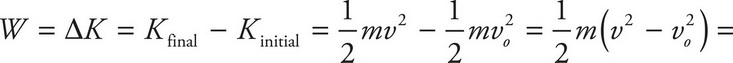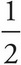# AP Physics 1 Question 390: Answer and Explanation

### Test Information

Question: 390

8. A student launched a small rocket with a mass of 50 kg into the air with an initial velocity of 10 m/s in the positive vertical direction. The student then turns on the secondary engines of the rocket to apply a constant upwards force that increases the velocity of the rocket to 15 m/s. If the force provided by the secondary engines did 4,500 J of work, how much work did the force of gravity do on the rocket?

• A. -625 J
• B. -750 J
• C. -1,250 J
• D. -1,375 J(50 kg)(15 m/s)2 - (10 m/s)2 =(50 kg)(225 m2/s2 - 100 m2/s2) = (25 kg)(125 m2/s2) = 3,125 J